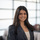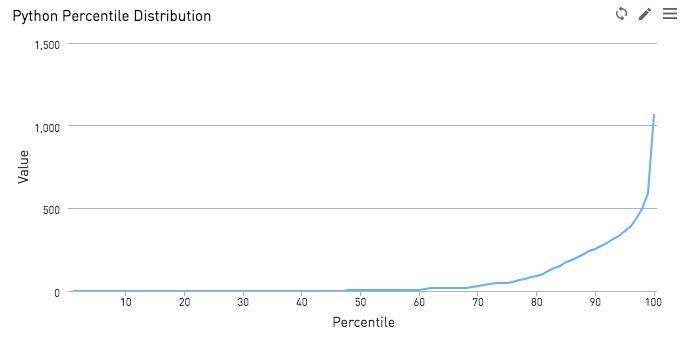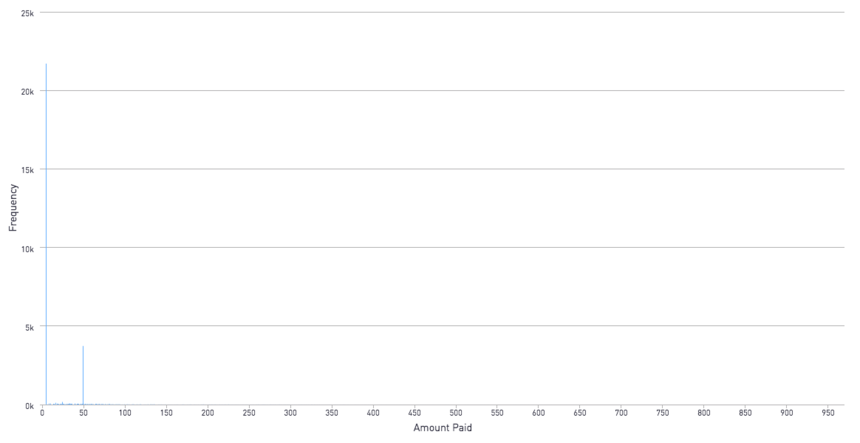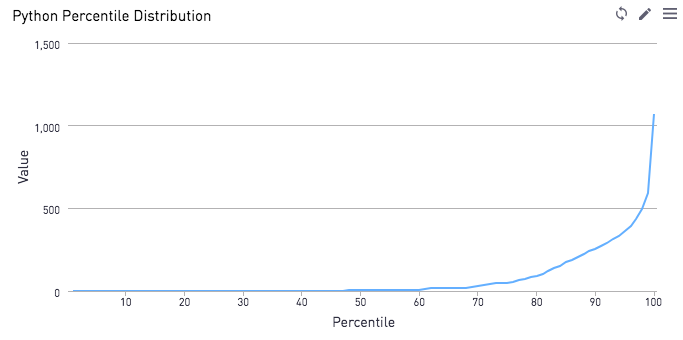# Percentile Curves - Another way to show distributionsNote: This solution requires Periscope's Python/R integration

Let's say you have data set that is heavily skewed, like this hypothetical data set here plotting the amount users paid on a fictional gaming app.As we can see here, a bar chart isn't a great way to visualize the data. Most users fall into the 99 cent bucket, so it is hard to see how many users fall into the other smaller buckets skewed to the right of the chart.

There's a couple ways we can re-display the data.

1. Via a histogram that buckets together certain ranges. But let's say we don't want to lose any granularity. Hop on down to option (2)
2. Plotting the percentile on the x axis, and the value that corresponds to the percentile on the y axis

Option (2) would look something like this:Now, we can easily see that well over half of users have paid 0.99 cents, and the higher paying customers make the top 20% of the data.

Here's the Python 3.6 code on how to get that information. Assume the information with the user ID and the amount they paid is stored in a dataframe called df.

```# SQL output is imported as a pandas dataframe variable called "df"
import pandas as pd
import matplotlib.pyplot as plt
from scipy.stats import scoreatpercentile

a=list(range(1,101))

b = [scoreatpercentile(df["amt_paid"],i) for i in a]

df2 = pd.DataFrame({'percentile': a, 'value': b}, columns=['percentile', 'value'])

# Use Periscope to visualize a dataframe, text, or an image by passing data to periscope.table(), periscope.text(), or periscope.image() respectively.
periscope.table(df2)```
3replies Oldest first
• Oldest first